# McGraw Hill Math Grade 8 Lesson 9.1 Answer Key Multiplying with Decimals

Practice the questions of McGraw Hill Math Grade 8 Answer Key PDF Lesson 9.1 Multiplying with Decimals to secure good marks & knowledge in the exams.

## McGraw-Hill Math Grade 8 Answer Key Lesson 9.1 Multiplying with Decimals

Exercises

MULTIPLY

Question 1.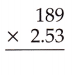478.17
Explanation:
while multiplying decimals ignore the decimals in the factors and multiply,
then count total number of decimal places in the factors.
Starting from the right of the product count the number of places,
then put the decimal point to the left of the last place.Question 2.17.45458
Explanation:
while multiplying decimals ignore the decimals in the factors and multiply,
then count total number of decimal places in the factors.
Starting from the right of the product count the number of places,
then put the decimal point to the left of the last place.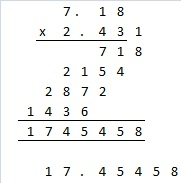Question 3.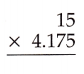62.625
Explanation:
while multiplying decimals ignore the decimals in the factors and multiply,
then count total number of decimal places in the factors.
Starting from the right of the product count the number of places,
then put the decimal point to the left of the last place.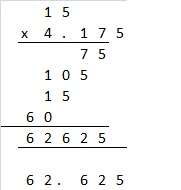Question 4.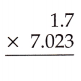11.9391
Explanation:
while multiplying decimals ignore the decimals in the factors and multiply,
then count total number of decimal places in the factors.
Starting from the right of the product count the number of places,
then put the decimal point to the left of the last place.Question 5.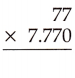598.29
Explanation:
while multiplying decimals ignore the decimals in the factors and multiply,
then count total number of decimal places in the factors.
Starting from the right of the product count the number of places,
then put the decimal point to the left of the last place.Question 6.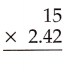36.3
Explanation:
while multiplying decimals ignore the decimals in the factors and multiply,
then count total number of decimal places in the factors.
Starting from the right of the product count the number of places,
then put the decimal point to the left of the last place.Question 7.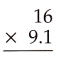145.6
Explanation:
while multiplying decimals ignore the decimals in the factors and multiply,
then count total number of decimal places in the factors.
Starting from the right of the product count the number of places,
then put the decimal point to the left of the last place.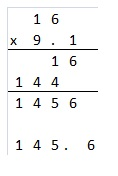Question 8.348.315
Explanation:
while multiplying decimals ignore the decimals in the factors and multiply,
then count total number of decimal places in the factors.
Starting from the right of the product count the number of places,
then put the decimal point to the left of the last place.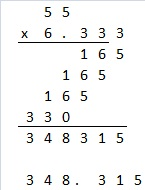Question 9.15107.4
Explanation:
while multiplying decimals ignore the decimals in the factors and multiply,
then count total number of decimal places in the factors.
Starting from the right of the product count the number of places,
then put the decimal point to the left of the last place.Question 10.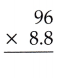844.8
Explanation:
while multiplying decimals ignore the decimals in the factors and multiply,
then count total number of decimal places in the factors.
Starting from the right of the product count the number of places,
then put the decimal point to the left of the last place.Question 11.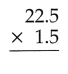33.75
Explanation:
while multiplying decimals ignore the decimals in the factors and multiply,
then count total number of decimal places in the factors.
Starting from the right of the product count the number of places,
then put the decimal point to the left of the last place.Question 12.12.88
Explanation:
while multiplying decimals ignore the decimals in the factors and multiply,
then count total number of decimal places in the factors.
Starting from the right of the product count the number of places,
then put the decimal point to the left of the last place.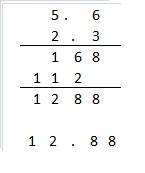Question 13.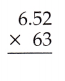410.76
Explanation:
while multiplying decimals ignore the decimals in the factors and multiply,
then count total number of decimal places in the factors.
Starting from the right of the product count the number of places,
then put the decimal point to the left of the last place.Question 14.5626.7379
Explanation:
while multiplying decimals ignore the decimals in the factors and multiply,
then count total number of decimal places in the factors.
Starting from the right of the product count the number of places,
then put the decimal point to the left of the last place.Question 15.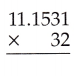356.8992
Explanation:
while multiplying decimals ignore the decimals in the factors and multiply,
then count total number of decimal places in the factors.
Starting from the right of the product count the number of places,
then put the decimal point to the left of the last place.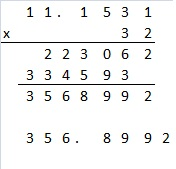Question 16.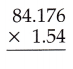129.63104
Explanation:
while multiplying decimals ignore the decimals in the factors and multiply,
then count total number of decimal places in the factors.
Starting from the right of the product count the number of places,
then put the decimal point to the left of the last place.129.63104

Question 17.
Pauline’s mom drives the soccer team to and from each of their away games. If the team has 12 away games, and on average Pauline’s mom uses 2.775 gallons of gas to make the round trip, how much gas will she use for the whole season?
33.3 gallons
Explanation:
If the team has 12 away games, and on average Pauline’s mom uses 2.775 gallons of gas to make the round trip,
Total gas will she use for the whole season 2.775 x 12 = 33.3 gallons

Question 18.
The delivery truck driver has 64 packages to deliver to the school. The average weight of a package is 13.7552 pounds. If the delivery truck has a load capacity of 900 pounds, can the delivery driver deliver all 64 packages in one load?